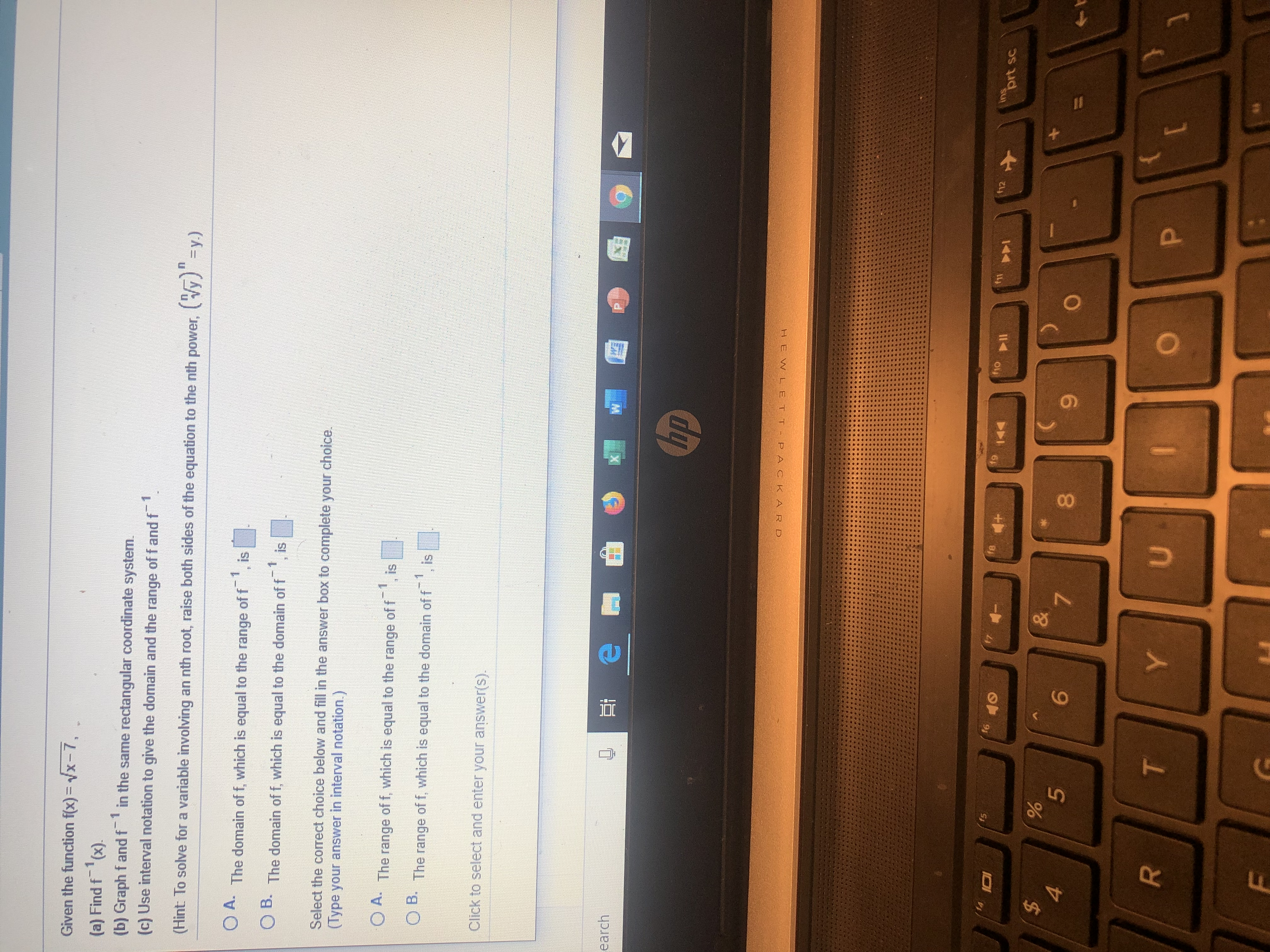# Given the function f(x) = vx-7,(a) Find f (x).(b) Graph f andf in the same rectangular coordinate system.(c) Use interval notation to give the domain and the range of f and f\$11(y)y.)(Hint To solve for a variable involving an nth root, raise both sides of the equation to the nth power,OA. The domain of f, which is equal to the range of fisOD. The domain of f, which is equal to the domain of fSelect the corect choice below and fill in the answer box to complete your choice.(Type your answer in interval notation.)1isOA The range of f, which is equal to the range of fB.The range of f, which is equal to the domain of fisClick to select and enter your answer(s).earchLhpHEWL E T T PACKA R Dinsprt sct12f9fufof6f5%5\$&76PYUF00TLL

Question
11 viewshelp_outlineImage TranscriptioncloseGiven the function f(x) = vx-7, (a) Find f (x). (b) Graph f andf in the same rectangular coordinate system. (c) Use interval notation to give the domain and the range of f and f \$1 1 (y)y.) (Hint To solve for a variable involving an nth root, raise both sides of the equation to the nth power, OA. The domain of f, which is equal to the range of f is OD. The domain of f, which is equal to the domain of f Select the corect choice below and fill in the answer box to complete your choice. (Type your answer in interval notation.) 1 is OA The range of f, which is equal to the range of f B. The range of f, which is equal to the domain of f is Click to select and enter your answer(s). earch Lhp HEWL E T T PACKA R D ins prt sc t12 f9 fu fo f6 f5 % 5 \$ & 7 6 P Y U F 00 T LL fullscreen
check_circle

Step 1

(a)

Consider the given function:

Step 2

Now, to find the inverse, solve for x in terms of y by squaring both sides:

Step 3

Now, replace y by x, and obtain the requ...

### Want to see the full answer?

See Solution

#### Want to see this answer and more?

Solutions are written by subject experts who are available 24/7. Questions are typically answered within 1 hour.*

See Solution
*Response times may vary by subject and question.
Tagged in

### Algebra